matlab
• 【实践】多条曲线在一幅图上，Origin如何对每一条曲线单独设置 双击图片的曲线，在弹出的设置窗口中找到Group中Edit Mode，将其设置成Independent。 点击左侧Graph1-->Layer1中的曲线，就可以单独设置了。 ...
    【实践】多条曲线在一幅图上，Origin如何对每一条曲线单独设置
双击图片的曲线，在弹出的设置窗口中找到Group中Edit Mode，将其设置成Independent。
点击左侧Graph1-->Layer1中的曲线，就可以单独设置了。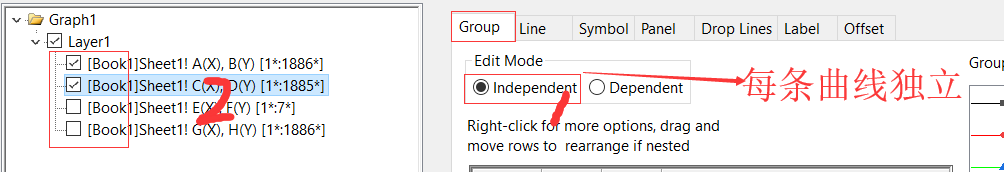展开全文• 如何判断一条曲线是否自己相交？area_holes
今天看到群里有人在问这个问题，想了一个解决办法。
我们首先作假设，如果一条曲线有交点，那么它就是相交的对吧。可能大家想的都是这样，就开始找各种方法去识别交点。
我们换个角度想一下：是不是我们判断这条曲线是否带有封闭的区域就可以了呢？
这样问题解决起来会很容易：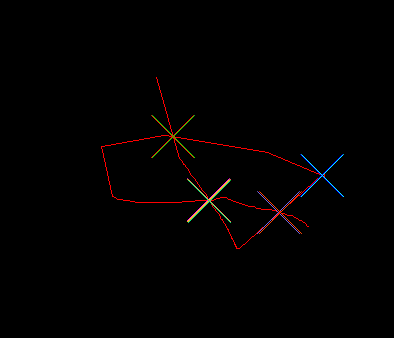图片里边有一条线，我先用求取交点做了一下。
如果采用求是否存在空洞的话程序会非常简单。
在halcon下实现：
首先选择出线条区域，可以用二值化。
threshold (Image, Region, 128, 255)
选择出区域后，可以填充一下孔洞，使用fill_up函数
fill_up (Region, RegionFillUp)
之后把没填充与填充的区域做减法
difference (RegionFillUp, Region, RegionDifference)
求取不同区域部分的面积
area_center (RegionDifference, Area, Row, Column)
如果Area大于0，则判定曲线有交点
进一步优化：
首先选择出线条区域，可以用二值化。
threshold (Image, Region, 128, 255)
直接判断是否存在孔洞
area_holes (RegionDifference, Area)
如果Area大于0，则判定曲线有交点
希望大家可以自己动手试一下


展开全文• 一张像素为480，270的bmp图片，其中有一条封闭的曲线，以图片中心点为原点，画一条射线，求射线每旋转一度，和封闭曲线的交点的像素点的坐标。求出360个点的坐标。
• ## 三次B样条曲线拟合算法

万次阅读 多人点赞 2017-01-17 22:10:28
三次B样条曲线方程B样条曲线分为近似拟合和插值拟合，所谓近似拟合就是不过特征点，而插值拟合就是通过特征点，但是插值拟合需要经过反算得到控制点再拟合出过特征点的B样条曲线方程。这里会次介绍两种拟合算法。...
1 三次B样条曲线方程
B样条曲线分为近似拟合和插值拟合，所谓近似拟合就是不过特征点，而插值拟合就是通过特征点，但是插值拟合需要经过反算得到控制点再拟合出过特征点的B样条曲线方程。这里会一次介绍两种拟合算法。首先介绍B样条的曲线方程。B样条曲线的总方程为：P(t)=∑i=0nPiFi,k(t)P(t)=\sum_{i=0}^{n} P_{i}F_{i,k}(t)    (1)
其中PiP_i是控制曲线的特征点，Fi,k(u)F_{i,k}(u)则是K阶B样条基函数。1.1 三次B样条曲线方程中基函数为：Fi,k(t)=1k!∑m=0k−i(−1)m(mk+1)(t+k−m−j)kF_{i,k}(t)=\frac{1}{k!}\sum_{m=0}^{k-i}(-1)^{m}\binom{m}{k+1}(t+k-m-j)^k  (2)
其中(mk+1)\binom{m}{k+1}表示阶乘，化成看的明白的式子就是：
（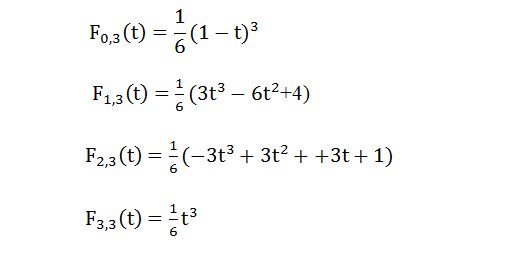将图片上的基函数代入到方程（1）中，就是：P(t)=P0∗F0,3(t)+P1∗F1,3(t)+P2∗F2,3(t)+P3∗F3,3(t)P(t)= P_0*F_{0,3}(t)+P_1*F_{1,3}(t)+P_2*F_{2,3}(t)+P_3*F_{3,3}(t)  (3)
方程（3）就是三次B样条曲线方程。

2019-04-18 更新

有小伙伴提到上式(2)的j是什么意思，其实j就是控制点的索引值。这里我把书上的公式摘抄下来，可能看起来更为清晰。
参考书籍：《计算机图形学 第3版》何援军 第13章三次B样条曲线计算F0,3(t)=13!∑j=03(−1)jC4j)(t+3−0−j)3F_{0,3}(t)=\frac{1}{3!}\sum_{j=0}^{3}(-1)^{j}C^{j}_{4})(t+3-0-j)^3
=16[(−1)0C40(t+3)3+(−1)1C41(t+2)3+(−1)2C42(t+1)3+(−1)3C43t3]=\frac{1}{6}[(-1)^{0}C^{0}_{4}(t+3)^{3}+(-1)^{1}C^{1}_{4}(t+2)^{3}+(-1)^{2} C^{2}_{4}(t+1)^{3}+(-1)^{3}C^{3}_{4}t^{3}]
=16(−t3+3t2−3t+1)=16(1−t)3=\frac{1}{6}(-t^{3}+3t^{2}-3t+1)=\frac{1}{6}(1-t)^{3}
F1,3(t)=13!∑j=02(−1)jC4j)(t+3−1−j)3F_{1,3}(t)=\frac{1}{3!}\sum_{j=0}^{2}(-1)^{j}C^{j}_{4})(t+3-1-j)^3
=16[(−1)0C40(t+2)3+(−1)1C41(t+1)3+(−1)2C42t3]=\frac{1}{6}[(-1)^{0}C^{0}_{4}(t+2)^{3}+(-1)^{1}C^{1}_{4}(t+1)^{3}+(-1)^{2} C^{2}_{4}t^{3}]
=16(3t3−6t2+4)=\frac{1}{6}(3t^{3}-6t^{2}+4)
F2,3(t)=13!∑j=01(−1)jC4j)(t+3−2−j)3F_{2,3}(t)=\frac{1}{3!}\sum_{j=0}^{1}(-1)^{j}C^{j}_{4})(t+3-2-j)^3
=16[(−1)0C40(t+1)3+(−1)1C41t3=\frac{1}{6}[(-1)^{0}C^{0}_{4}(t+1)^{3}+(-1)^{1}C^{1}_{4}t^{3}
=16(−3t3+3t2+3t+1)=\frac{1}{6}(-3t^{3}+3t^{2}+3t+1)
F3,3(t)=13!∑j=00(−1)jC4j)(t+3−3−j)3F_{3,3}(t)=\frac{1}{3!}\sum_{j=0}^{0}(-1)^{j}C^{j}_{4})(t+3-3-j)^3
=16[(−1)0C40t3=\frac{1}{6}[(-1)^{0}C^{0}_{4}t^{3}
=16t3=\frac{1}{6}t^{3}
2 三次B样条曲线近似拟合
近似拟合很简单。不需要求控制点，求得Fi,k(t)F_{i,k}(t)，由上述方程（3），代入P0,P1,P2,P3P_0,P_1,P_2,P_3就可以得到由这四个点近似拟合的一段三次B样条曲线，起始点在P0P_0，终点在P1P_1，对于闭合轮廓，最后一段可以取前两点做辅助，拟合实验结果我最后一块给出。这种近似拟合曲线光滑，但是最大不足就是不过特征点，也就是不过PiP_i，需要过点需要反求控制点再拟合。
3 三次B样条插值拟合
插值拟合较为复杂。其实也不算是很复杂，找资料过程和理解过程是一个复杂的过程。不过有了前面大神做工作，我们只是借用别人的成果写代码就好了。我给大家看一篇论文，大家可以百度或者去知网搜索，闭合 B 样条曲线控制点的快速求解算法及应用。文章讲解了反求控制点的具体步骤，写的非常详细，基本上贴近代码的那种。大家可以根据这篇论文反求控制点，拟合出来的三次B样条曲线是经过PiP_i的。代码就不放了，很多，可以根据我给的那篇论文直接编写相应代码，有问题可以私信我，知无不言。
##4 拟合结果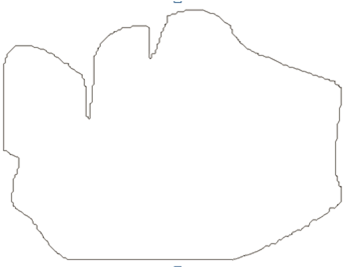原轮廓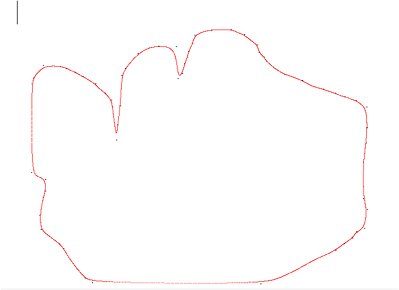近似拟合轮廓。可以看到没过黑色特征点，只是近似拟合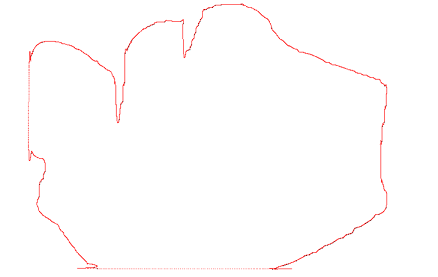插值拟。可以看到曲线经过黑色特征点，不过有一些不足之处。
##5 总结
三次B样条曲线拟合轮廓效果还是可以，较之Beizer（可以参考我博客三次Beizer曲线拟合算法），B样条将一些细节描述的很好，很多细节之处都贴近原轮廓，但是有一些不足之处，可以看到对直线拟合效果不是很好。两篇博客都是关于闭合轮廓的拟合，对于非闭合或者只是一段曲线拟合，还有一种曲线是很好的，《数值分析》提到过，叫三次样条插值拟合，拟合效果很好，我做过拟合一元三次方程曲线，拟合效果跟原曲线非常贴近，不过过程中需要用到追赶法，而追赶法需要满足一个条件，对于闭合曲线三次样条插值是不满足这个条件的，所以我没去深研究，大家可以去试一试。谢谢大家！
-------------------------------------------------2018-10-30--------------------------------------------
真的很感谢大家的支持，这一年都比较忙，找实习and找工作，而且这个东西是研一上学期搞的，现在看都毫无印象，对自己说一句：牛逼。我把代码放在网盘了（能运行，但现在没效果，大家可以检查下，之前是OK的），本来想上传到CSDN，好像要C币，而且要审核，太墨迹了。
链接: https://pan.baidu.com/s/1mSQMmvL71gwEAqgiT6O9Gg 提取码: xv5f


展开全文openCV 轮廓拟合
• 贝塞尔曲线 基本公式：B(t)=∑i=0n(in)Pi(1−t)n−iti，t∈[0,1]基本公式：B(t)=\sum_{i=0}^{n} \Big({_i^n}\Big)P_i(1-t)^{n-i}t^i，t\in[0,1]基本公式：B(t)=i=0∑n​(in​)Pi​(1−t)n−iti，t∈[0,1] 三次...
贝塞尔曲线
$基本公式：B(t)=\sum_{i=0}^{n} \Big({_i^n}\Big)P_i(1-t)^{n-i}t^i，t\in[0,1]$
三次贝塞尔曲线：
$B(t)=P_0(1-t)^3+3P_1t(1-t)^2+3P_2t^2(1-t)+P_3t^3，t\in[0,1]$
由此可见其系数规律：
$1\ 1$$1\ 2\ 1$$1\ 3\ 3\ 1$$1\ 4\ 6\ 4\ 1$
分别为一阶到四阶的系数规律，变化规律为杨辉三角，并且$t$与$(t-1)$的规律是一个逐渐转变的一个过程。
一段贝塞尔曲线拟合程序：
import matplotlib.pyplot as plt

x = [0, 1, 2, 3, 4, 5, 6, 7, 8, 9]
y = [12, 2, 78, 12, 34, 23, 67, 87, 98, 10]
xnew = []
ynew = []

def Bazier_3(m1, m2):
for i in range(101):
t = i / 100
xnew.append(m1 * (1 - t) ** 3 + 3 * m1 * t * (1 - t) ** 2 + 3 * m1 * t ** 2 * (1 - t) + m1 * t ** 3)
ynew.append(m2 * (1 - t) ** 3 + 3 * m2 * t * (1 - t) ** 2 + 3 * m2 * t ** 2 * (1 - t) + m2 * t ** 3)

for i in range(len(x) // 3):
Bazier_3(x[i * 3:(i + 1) * 3 + 1], y[i * 3:(i + 1) * 3 + 1])

plt.plot(xnew, ynew)
plt.plot(x, y)
plt.scatter(x, y)
plt.show()

可以看到 ，在3，6这两个点，并不满族c2连续。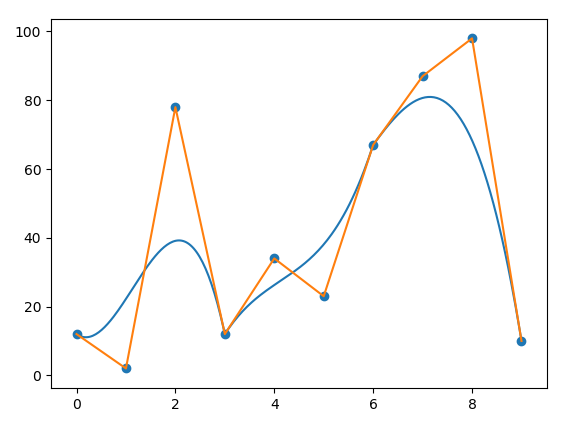B样条曲线
$基本公式：\vec{P}(t)=\sum_{i=0}^{n}\vec{P_{i}}B_{i,n}(t)$
其基本形式与贝塞尔曲线相似
$其中T=[t_{0},t_{1},t_{2},...,t_{n}]$
$N_{i,0}(t)=\Big \{ ^{0\qquad\qquad t_{i}{\le}t{\le}t_{i+1}}_{1\qquad\qquad t>t_{i}或t{\ge}t_{i+1}}$
$N_{i,k}(t)=\frac{t-t_i}{t_{i+k}-t_i}N_{i,k-1}(t)+\frac{t_{i+k+1}-t}{t_{i+k+1}-t_{i+n}}N_{i+1,k-1}(t)\qquad k\ge1$
$其中，N_{i,k}(t)中的i是控制点，k是次数$
用的最多的是三次B样条曲线。
其中：
$N_{0,3}(t)=\frac{1}{6}(-t^3+3t^2-3t+1)$
$N_{1,3}(t)=\frac{1}{6}(3t^3-6t^2+4)$
$N_{2,3}(t)=\frac{1}{6}(-3t^3-3t^2+3t+1)$
$N_{1,3}(t)=\frac{1}{6}t^3$
为了使其闭合，要取最后一个点与与第一个控制点相同，即$P_{m+1}=P_0$，$P_{m+2}=P_1$，$P_{m+3}=P_2$，这样的曲线满足$c_2$连续。
一个拼接示例：
import matplotlib.pyplot as plt

x = [0, 1, 2, 3, 4, 5, 6, 7, 8, 9]
y = [12, 2, 78, 12, 34, 23, 67, 87, 98, 10]
xnew = []
ynew = []

arg = [[-1, 3, -3, 1], [3, -6, 0, 4], [-3, 3, 3, 1], [1, 0, 0, 0]]

def Ba(t, coefficient):
return (coefficient * t ** 3 + coefficient * t ** 2 + coefficient * t + coefficient) / 6

def creat(n):
for i in range(101):
t = i / 100
xnew.append(
x[n + 0] * Ba(t, arg) + x[n + 1] * Ba(t, arg) + x[n + 2] * Ba(t, arg) + x[n + 3] * Ba(t, arg))
ynew.append(
y[n + 0] * Ba(t, arg) + y[n + 1] * Ba(t, arg) + y[n + 2] * Ba(t, arg) + y[n + 3] * Ba(t, arg))

for i in range(7):
creat(i)

plt.plot(xnew, ynew)
plt.plot(x, y)
plt.scatter(x, y)
plt.show()

拼接后的图片如下所示，满足$c_2$连续。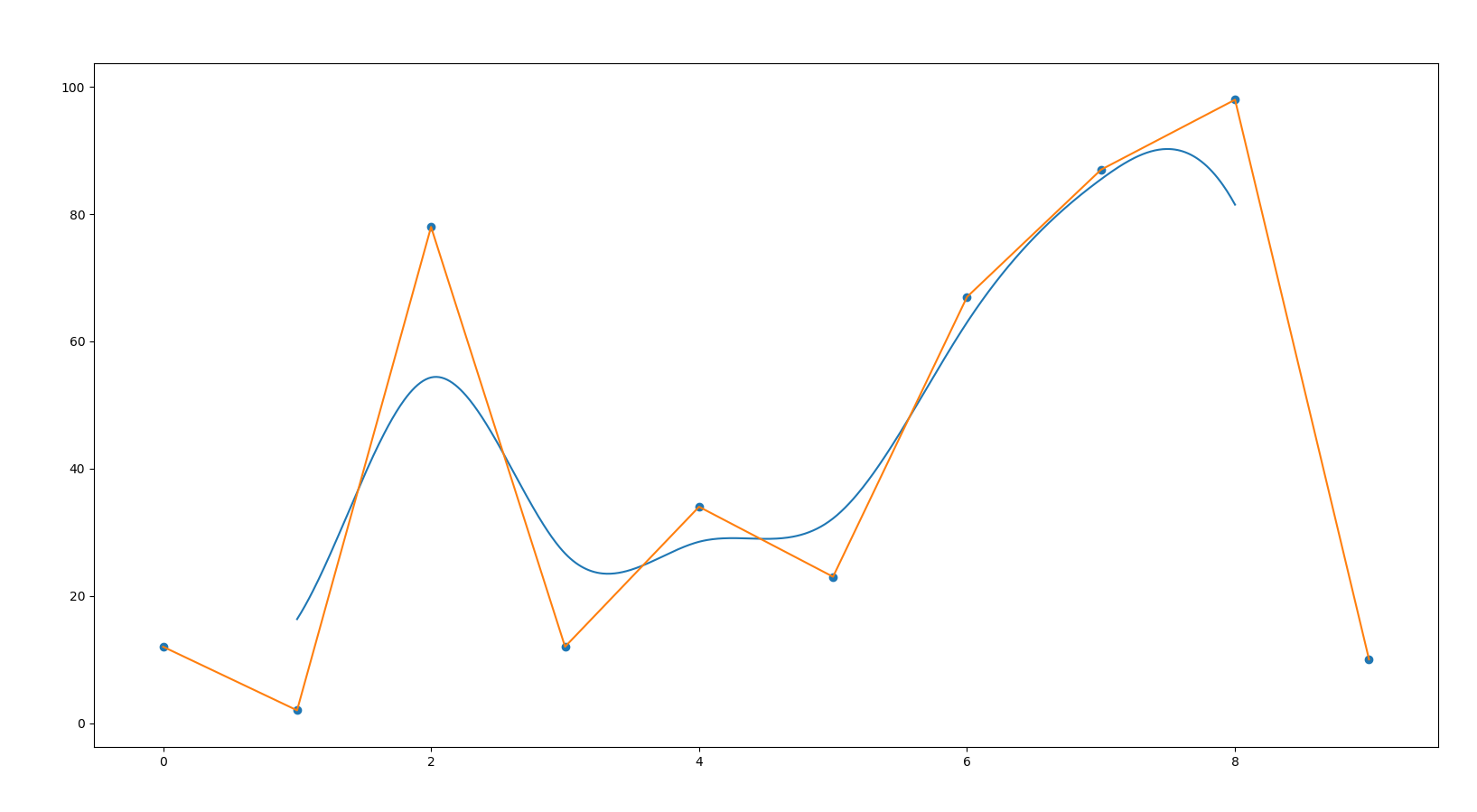贝塞尔曲线与B样条曲线的结合：
import matplotlib.pyplot as plt
import numpy as np
from mpl_toolkits.mplot3d import Axes3D

arg = [[-1, 3, -3, 1], [3, -6, 0, 4], [-3, 3, 3, 1], [1, 0, 0, 0]]  # B样条曲线的拟合参数
mar = [[0, -93.70105743, -7.71050644, 11.30164337],
[0, -81.51637268, 2.65858841, 21.85936928],
[0, -100.43165588, -506.84307861, -277.47509766],
[0, -413.89691162, -244.77906799, -228.4907074],
[0, -74.61241913, 22.23334312, 28.80702591],
[0, -57.65986252, 33.13808441, 40.01465988],
[0, -23.39715576, -46.36453247, -68.40002441],
[0, -427.99655151, -242.35075378, -246.75854492],
[0, -93.70105743, -7.71050644, 11.30164337]]  # 原数据

def Bazier_3(m1, m2):  # 贝塞尔曲线拟合
x = []
y = []
for i in range(101):
t = i / 100
x.append(m1 * (1 - t) ** 3 + 3 * m1 * t * (1 - t) ** 2 + 3 * m1 * t ** 2 * (1 - t) + m1 * t ** 3)
y.append(m2 * (1 - t) ** 3 + 3 * m2 * t * (1 - t) ** 2 + 3 * m2 * t ** 2 * (1 - t) + m2 * t ** 3)
return x, y

def Ba(t, coefficient):  # 参数合成
return (coefficient * t ** 3 + coefficient * t ** 2 + coefficient * t + coefficient) / 6

def creat_mart(mart):  # 贝塞尔曲线生成
re = []
for i in range(len(mart)):
temp_x, temp_y = Bazier_3([0, 1, 2, 3], mart[i])
re.append(temp_y)
return re, temp_x

def creat_mart_finnally(data):  # 最终生成
out = []
times = data.shape
for j in range(times - 1):
for i in range(45):
t = i / 45
temp = 0
for k in range(4):
temp += data[(j + k) % times] * Ba(t, arg[k])
out.append(temp)
return out

def draw(mat1, mat2, mat3):
x = np.linspace(0, 8, 9)
y = np.linspace(0, 3, 4)
x, y = np.meshgrid(x, y)
x = x.T
y = y.T
xs = np.ravel(x)
ys = np.ravel(y)
zs = np.ravel(mat1)

xnew = np.linspace(0, 8, 360)
ynew = np.linspace(0, 3, 101)
xn, yn = np.meshgrid(xnew, ynew)

x_min = np.linspace(0, 8, 9)
y_min = np.linspace(0, 3, 101)
x_min, y_min = np.meshgrid(x_min, y_min)

plt.figure("原数据")
ax = plt.subplot(1, 1, 1, projection='3d')
ax.plot_trisurf(xs, ys, zs, cmap='coolwarm')
ax.set_xlabel('angle')
ax.set_ylabel('stepsize')
ax.set_zlabel('Z')
plt.title('raw')

plt.figure("最终数据")
ax2 = plt.subplot(1, 1, 1, projection='3d')
ax2.plot_surface(xn.T, yn.T, mat2, rstride=2, cstride=2, cmap='coolwarm', linewidth=0.5, antialiased=True)
ax2.set_xlabel('angle')
ax2.set_ylabel('stepsize')
ax2.set_zlabel('Z')
plt.title('processed')

plt.figure("中间数据")
ax3 = plt.subplot(1, 1, 1, projection='3d')
ax3.plot_surface(x_min.T, y_min.T, mat3, rstride=2, cstride=2, cmap='coolwarm', linewidth=0.5, antialiased=True)
ax3.set_xlabel('angle')
ax3.set_ylabel('stepsize')
ax3.set_zlabel('Z')
plt.title('min')

plt.show()

mat_new, _ = creat_mart(mar)

mat_new = np.array(mat_new)

mat_f = []
for i in range(mat_new.shape):
mat_f.append(creat_mart_finnally(mat_new[:, i]))

mat_f = np.array(mat_f).T

draw(np.array(mar), mat_f, mat_new)

生成的图片如下：
源数据：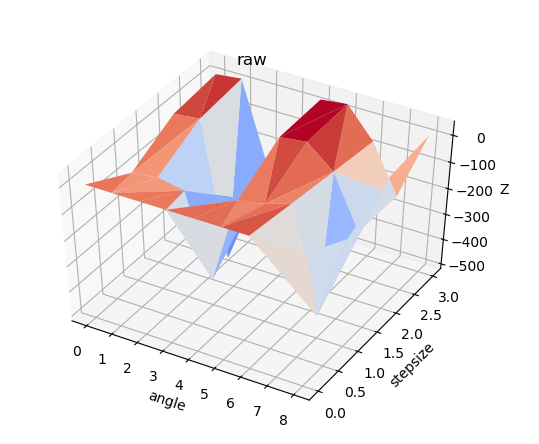在步长方向上进行了贝塞尔曲线插值之后的数据是这样的：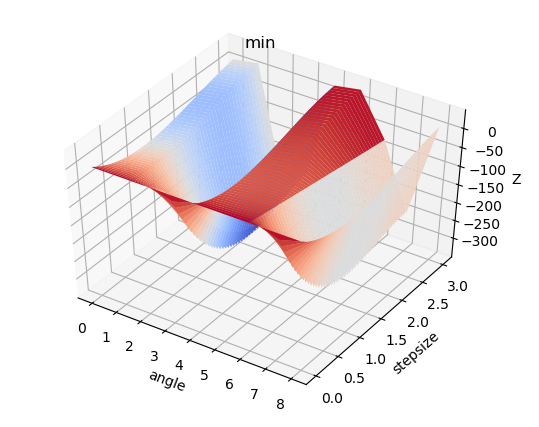在角度方向上面进行了B样条曲线插值：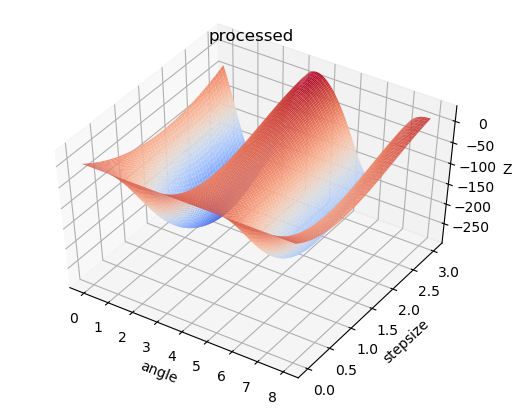展开全文贝塞尔曲线
• %第列的所有数据 若是仅仅画数据，直接用plot(A) 例子如下： filename = 'I.jpg'; imgData = imread(filename); imshow(imgData);%该函数可以用来显示已经读入的数据 A = imgData(:...matlab
• 目的：提取图片上的曲线数据点。比如，文献中的图表数据。 源代码 function varargout = DataExtract(varargin) % DATAEXTRACT MATLAB code for DataExtract.fig % DATAEXTRACT, by itself, creates a new DATA...
• 如上图所示，我希望求这个较粗曲线每隔段的个中点，结果大概是这样： [img=https://img-bbs.csdn.net/upload/201705/31/1496200413_250690.jpg][/img] 该怎么实现? 我之前尝试过先提取骨架 再找点 效果不错...opencv
• #第二条曲线 x = range(0, len(y1)) plt.plot(x, y1, color='red', label='$M_1$', linewidth=0.8) plt.legend(loc='upper right', prop=font1, frameon=False) # 绘制图例，指定图例位置 plt.xticks([]) # 去掉x...
• ## TensorBoard一幅图中显示多条曲线

万次阅读 热门讨论 2018-03-02 15:10:33
from numpy import random writer_1 = tf.summary.FileWriter("./logs/plot_1") writer_2 = tf.summary.FileWriter("./logs/plot_2") log_var = tf.Variable(0.0) tf.summary.s...
• 文章中的图片上有多个曲线，数据已经丢失，想要图片上的个周期的数据。 这个图片分两种： 1，已经转化成图片了，图片格式的，没办法，只能步步解决。 2，另种从别的软件里拷贝出来直接粘贴到word中，还未转化...matlab
• ## OpenCV 提取图片中的曲线

万次阅读 多人点赞 2017-05-07 14:23:46
首先，输入的图片是一张二值图片 (白色为曲线)，其中包含的曲线宽度为 1 像素的 (如果曲线不是 1 像素的 先提取其骨架)。遍历寻找图像中第个白色的点，然后从这个点开始延伸寻找曲线。注意，第个找opencv 图像处理
• 2，在一张图中显示多条曲线 3，在个窗口显示多张图片 注意： 1，问题背景 在次任务中，老师给出了几个公式，其中含有三个自变量λ、μ、c。因为要观察最终的结果受这三个变量的影响，所以采用如下的展示方式...python plot画图
• 然后用白色画笔覆盖，留一条曲线出来就行。第二步，打开getdata软件。第三步，打开图像。第四步，设置坐标。第五步，取出数据。第六步 导出数据 可以用getdata自带的，麻烦。提倡直接鼠标拉取想要的数据。拉取选好后...
•  跟着我的思路往下走（f也是这样一步一步去画的）首先画出一条贝塞尔曲线，然后控制曲线旋转角度不断变化，视觉上就感觉是一个中心固定的几条贝塞尔曲线在旋转，也可以同时画出几条贝塞尔曲线，控制旋转来达到类似...android
• 目录0.引言1.思路详解与分析2.MATLAB程序 0.引言   在读文献的时，经常遇到这样的情况：文章里提出的方法...1.1准备待提取数据的曲线图片 将待提取数据的曲线的图片(如.jpg格式图片)，利用 imread 输入到 matlamatlab
• Highcharts 是个用纯JavaScript编写的个图表库，在web前端展示中可以做出很炫的图表，...但又有一些相似之处，如都是XY型（时间为X），都包含基线（多条曲线）等，基于这种特征，需要写个通用的接口，方便多指
• 做数据分析，还有机器学习的收敛性，准确性分析时，往往需要将一些数据图形化，以曲线的形式显示出来，下面就介绍两种方式来实现这个小问题，种是object-oriented面向对象的，另种是基于plt的。 下面这个方式是...
• 在这章节，我们以其中曲线段为例，改变其对应的控制点，看看曲线段形状的改变，同时也看看对应的回旋体图形的改变。 控制点坐标如下图： “问题六十”中的“基本曲线”的控制点对应如上ABCDEF六个点C++
• 虽然有些东西似乎是显然的，但个完整的定义仍然很有必要。比如，大多数人并不知道函数的连续性是怎么定义的，虽然大家一直在用。有人可能会说，函数是不是连续的看就知道了嘛，需要定义么。事实上，如果没有严格...matrix 联想 扩展 c
• 在matplotlib 中，个figure即为个画板，用plt.figure()创建个新画板，如果只有个画板的话这句可以省略。 #创建第个视图（画板） plt.figure(1) #第个画板的内容 #… #创建第二个视图（画板） plt.figure...python 数据可视化 可视化
• 步：打开Grapher，由散点生成曲线 第二步：曲线打印生成图片 第三步，打开GetData软件，打开图片 第四步：SetScale，指定Xmin，Xmax，Ymin，Ymax 第五步：Digitalize Area, 1）指定X间隔，比如5m ...
• 转载自：https://blog.csdn.net/auto1993/article/details/71297151从上面的这个博客里面看到个用Python调用网络的代码，然后就试了试，发现了发现不能直接跑通，因此这个博客就主要介绍下这个代码怎么修改。...caffe
• GateData Graph Digitizrer介绍获取图片曲线数据提取 介绍 首先先来介绍款科研利器GateData Graph Digitizrer 这款软件可以将论文、或者纸质的图表数据提取出来，其原理是根据像素分析，首先设定图片中的坐标轴，...
•    GDI+的矢量绘图部分被用来绘制线条、绘制曲线和填充图形。... <br />电脑显示器在个矩形点阵(即分辨率)上创建其显示画面，这些点称为图片要素或者象素。不同的显示器其在屏幕microsoft vector windows...